SBOS688A April   2015  – October 2015

PRODUCTION DATA.

1. Features
2. Applications
3. Description
4. Revision History
5. Pin Configuration and Functions
6. Specifications
7. Parameter Measurement Information
8. Detailed Description
1. 8.1 Overview
2. 8.2 Functional Block Diagram
3. 8.3 Feature Description
4. 8.4 Device Functional Modes
9. Application and Implementation
1. 9.1 Application Information
2. 9.2 Typical Applications
1. 9.2.1 Single-Supply, 16-Bit, 1-MSPS SAR ADC Driver
2. 9.2.2 Single-Supply, 16-Bit, 1-MSPS, Multiplexed, SAR ADC Driver
10. 10Power Supply Recommendations
11. 11Layout
12. 12Device and Documentation Support
1. 12.1 Device Support
1. 12.1.1 Development Support
2. 12.2 Documentation Support
4. 12.4 Community Resources
6. 12.6 Electrostatic Discharge Caution
7. 12.7 Glossary
13. 13Mechanical, Packaging, and Orderable Information

• DBV|6

## 6 Specifications

### 6.1 Absolute Maximum Ratings

over operating free-air temperature range (unless otherwise noted)(1)
MIN MAX UNIT
Supply voltage, VS (V+) – (V–) 6 V
Input voltage(2) +IN (V–) – 0.3 (V+) + 0.3 V
–IN (V–) – 0.3 (V+) + 0.3
MODE (V–) – 0.3 (V+) + 0.3
Output voltage OUT (V–) (V+) V
Sink current +IN 10 mA
–IN 10
MODE 10
OUT 150
Source current +IN 10 mA
–IN 10
MODE 10
OUT(2) 150
Temperature Operating junction –40 150 °C
Storage, Tstg –65 150
(1) Stresses beyond those listed under Absolute Maximum Ratings may cause permanent damage to the device. These are stress ratings only, which do not imply functional operation of the device at these or any other conditions beyond those indicated under Recommended Operating Conditions. Exposure to absolute-maximum-rated conditions for extended periods may affect device reliability.
(2) For input voltages beyond the power-supply rails, voltage or current must be limited.

### 6.2 ESD Ratings

VALUE UNIT
V(ESD) Electrostatic discharge Human-body model (HBM), per ANSI/ESDA/JEDEC JS-001(1) ±3000 V
Charged-device model (CDM), per JEDEC specification JESD22-C101(2) ±1500
(1) JEDEC document JEP155 states that 500-V HBM allows safe manufacturing with a standard ESD control process.
(2) JEDEC document JEP157 states that 250-V CDM allows safe manufacturing with a standard ESD control process.

### 6.3 Recommended Operating Conditions

over operating free-air temperature range (unless otherwise noted)
MIN NOM MAX UNIT
VS Supply input voltage, (V+) – (V–) 2.7 5.5 V
VI Input voltage +IN (V–) (V+) – 1.15 V
–IN (V–) (V+) – 1.15
MODE (V–) (V+)
VO Output voltage (V–) (V+) V
IO Output current –120 120 mA
TA Operating free-air temperature –40 125 °C
TJ Operating junction temperature –40 125 °C

### 6.4 Thermal Information

THERMAL METRIC(1) OPA625 OPA2625 UNIT
DBV (SOT) DGS (VSSOP)
6 PINS 10 PINS
RθJA Junction-to-ambient thermal resistance 184.9 171.7 °C/W
RθJC(top) Junction-to-case (top) thermal resistance 123.6 68.4 °C/W
RθJB Junction-to-board thermal resistance 30.7 91.9 °C/W
ψJT Junction-to-top characterization parameter 22.1 9.4 °C/W
ψJB Junction-to-board characterization parameter 30.2 90.5 °C/W
RθJC(bot) Junction-to-case (bottom) thermal resistance N/A N/A °C/W

### 6.5 Electrical Characteristics High-Drive Mode

at TA = 25°C, V+ = 5 V, V– = 0 V, MODE pin connected to V– pin, VCOM = VO = 2.5 V, gain (G) = 1, RF = 1 kΩ, CF = 2.7 pF, CLOAD = 20 pF, and RLOAD = 2 kΩ connected to 2.5 V (unless otherwise noted)
PARAMETER TEST CONDITIONS MIN TYP MAX UNIT
AC PERFORMANCE
Unity gain frequency VO = 10 mVPP 80 MHz
φm Phase margin 50 Degrees
GBW Gain-bandwidth product G = 100, VO = 10 mVPP 120 MHz
SR Slew rate VO = 1-V step, G = 1 45 V/µs
VO = 4-V step, G = 2 115
tsettle Settling time VO = 4-V step, G = 2 Settling time to 0.1%
(10-bit accuracy)
80 ns
to 0.005%
(14-bit accuracy)
110
to 0.00153%
(16-bit accuracy)
280
Overshoot VO = 4-V step, G = 2 2.5%
Undershoot VO = 4-V step, G = 2 3%
HD2 Second-order harmonic Distortion VO = 2 VPP, G = 2 f = 10 kHz 144 dBc
f = 100 kHz 122
f = 1 MHz 80
HD3 Third-order harmonic Distortion VO = 2 VPP, G = 2 f = 10 kHz 155 dBc
f = 100 kHz 140
f = 1 MHz 80
Second-order intermodulation distortion VO = 2 VPP, f = 1 MHz, 200-kHz tone spacing 90 dBc
Third-order intermodulation distortion VO = 2 VPP, f = 1 MHz, 200-kHz tone spacing 100 dBc
VN Input noise voltage f = 0.1 Hz to 10 Hz, peak-to-peak 0.8 µVPP
f = 0.1 Hz to 10 Hz, rms 120 nVRMS
Vn Input voltage noise density f = 1 kHz 3.2 nV/√Hz
f = 10 kHz 2.5
In Input current noise density f = 1 kHz 4.1 pA/√Hz
f = 10 kHz 2.8
tOR Overload recovery time G = 5 50 ns
Zo Open-loop output impedance f = 1 MHz 1 Ω
Crosstalk DC 150 dB
f = 1 MHz 127
DC PERFORMANCE
VOS Input offset voltage 15 ±100 µV
TA = –40°C to +125°C ±300
dVOS/dT Input offset voltage drift TA = –40°C to +125°C 0.5 ±3 µV/°C
OPA2625 only, TA = –40°C to +125°C 0.6 ±4
PSRR Power-supply rejection ratio 2.7 V ≤ (V+) ≤ 5 V 100 dB
TA = –40°C to +125°C 90 120
IB Input bias current 2 4 µA
TA = –40°C to +125°C 5.7
OPA2625 only, TA = –40°C to +125°C 6.5
dIB/dT Input bias current drift TA = –40°C to +125°C 15 nA/°C
IOS Input offset current 20 120 nA
TA = –40°C to +125°C 150
OPA2625 only, TA = –40°C to +125°C 200
dIOS/dT Input offset current drift TA = –40°C to +125°C 0.6 nA/°C
OPEN LOOP GAIN
AOL Open-loop gain (V–) + 0.2 V < VO < (V+) – 0.2 V, RLOAD = 600 Ω 110 dB
(V–) + 0.15 V < VO < (V+) – 0.15 V, RLOAD = 10 kΩ 114
TA = –40°C to +125°C (V–) + 0.2 V < VO < (V+) – 0.2 V,
106 128
(V–) + 0.15 V < VO < (V+) – 0.15 V,
110 132
INPUT VOLTAGE
VCM Common-mode voltage range TA = –40°C to +125°C (V–) (V+) – 1.15 V
CMRR Common-mode rejection ratio (V–) < VCOM < (V+) – 1.15 V 100 117 dB
TA = –40°C to +125°C 90 115
INPUT IMPEDANCE
ZID Differential input impedance 27 || 1.2 KΩ || pF
ZIC Common-mode input impedance 47 || 1.5 MΩ || pF
OUTPUT
Output voltage swing to the rail RLOAD = 600 Ω 60 80 mV
TA = –40°C to +125°C 100
RLOAD = 10 kΩ 20 35
TA = –40°C to +125°C 40
Isc Short-circuit current 150 mA
MODE
VIL High-drive (HD) mode threshold TA = –40°C to +125°C (V–) (V–) + 0.5 V
VIH Low-power (LP) mode threshold TA = –40°C to +125°C (V–) + 1.2 (V+) V
IIL Low-level input current TA = –40°C to +125°C, VMODE ≤ (V–) + 0.5 V 0.01 1 µA
IIH High-level input current TA = –40°C to +125°C, VMODE ≥ (V–) + 1.2 V 20 30 µA
OPA2625 only, TA = –40°C to +125°C, VMODE ≥ (V–) + 1.2 V 1
POWER SUPPLY
IQ Quiescent current per amplifier IO = 0 mA,
MODE connected to ground
2 2.2 mA
TA = –40°C to +125°C 3.1

### 6.6 Electrical Characteristics Low-Power Mode

at TA = 25°C, V+ = 5 V, V– = 0 V, VMODE = 5 V, VCOM = VO = 2.5 V, gain (G) = 1, RF = 1 kΩ, CF = 2.7 pF, CLOAD = 20 pF, and RLOAD = 2 kΩ connected to 2.5 V (unless otherwise noted)
PARAMETER TEST CONDITIONS MIN TYP MAX UNIT
AC PERFORMANCE
GBW Gain-bandwidth product G = 100, VO = 10 mVPP 1 MHz
φm Phase margin 72 Degrees
SR Slew rate VO = 1-V step 4.3 V/µs
VO = 4-V step, G = 2 4.1
Zo Open-loop output impedance f = 1 MHz 12 Ω
DC PERFORMANCE
VOS Input offset voltage 0.6 3 mV
TA = –40°C to +125°C 0.7 3.7
PSRR Power-supply rejection ratio 2.7 V ≤ (V+) ≤ 5 V 74 dB
TA = –40°C to +125°C 70 100
IB Input bias current 150 nA
TA = –40°C to +125°C 140 200
OPA2625 only, TA = –40°C to +125°C 250
IOS Input offset current 20 nA
TA = –40°C to +125°C 25
OPEN LOOP GAIN
AOL Open-loop gain TA = –40°C to +125°C (V–) + 0.2 V < VO < (V+) – 0.2 V,
70 100 dB
(V–) + 0.15 V < VO < (V+) – 0.15 V,
90 100
INPUT VOLTAGE
VCM Common-mode voltage range TA = –40°C to +125°C (V–) (V+) – 1.15 V
CMRR Common-mode rejection ratio (V–) < VCOM < (V+) – 1.15 V 66 114 dB
TA = –40°C to +125°C 60 114
OUTPUT
Output voltage swing to the rail TA = –40°C to +125°C RLOAD = 600 Ω 110 mV
Isc Short-circuit current 100 mA
POWER SUPPLY
IQ Quiescent current per amplifier IO = 0 mA,
MODE connected to V+
270 320 µA
TA = –40°C to +125°C 450

### 6.7 Electrical Characteristics High-Drive Mode

at TA = +25°C, V+ = 2.7 V, V– = 0 V, VMODE = 0 V, VCOM = VO = 1.35 V, gain (G) = 1, RF = 1 kΩ, CF = 2.7 pF, CLOAD = 20 pF, and RLOAD = 1 kΩ connected to 1.35 V (unless otherwise noted)
PARAMETER TEST CONDITIONS MIN TYP MAX UNIT
AC PERFORMANCE
Unity gain frequency VO = 10 mVPP 76 MHz
φm Phase margin 45 Degrees
GBW Gain-bandwidth product G = 100, VO = 10 mVPP 120 MHz
SR Slew rate VO = 1-V step, G = 2 45 V/µs
tsettle Settling time VO = 1-V step, G = 2 to 0.1% 80 ns
to 0.01% 170
to 0.000763% (17-bit accuracy) 250
Overshoot VO = 1-V step, G = 2 6%
Undershoot VO = 1-V step, G = 2 5%
HD2 Second order harmonic Distortion (V+) = 3.3 V, (V–) = 0 V, VCOM = 1.1 V,
VO = 2 VPP
f = 10 kHz 136 dBc
f = 100 kHz 118
f = 1 MHz 80
HD3 Third order harmonic Distortion (V+) = 3.3 V, (V–) = 0 V, VCOM = 1.1 V,
VO = 2 VPP
f = 10 kHz 143 dBc
OPA2625 only, f = 10 kHz 143
f = 100 kHz 130
OPA2625 only, f = 100 kHz 125
f = 1 MHz 85
OPA2625 only, f = 1 MHz 74
Second order inter-modulation distortion (V+) = 3.3 V, (V–) = 0 V, VCOM = 1.1 V, VO = 2 VPP,
f = 1 MHz, 200-kHz tone spacing
95 dBc
Third order inter-modulation distortion (V+) = 3.3 V, (V–) = 0 V, VCOM = 1.1V, VO = 1 VPP,
f = 1 MHz, 200-kHz tone spacing
104 dBc
VN Input noise voltage f = 0.1 Hz to 10 Hz peak to peak 0.8 µVPP
f = 0.1 Hz to 10 Hz rms 120 nVRMS
Vn Input voltage noise density f = 10 kHz 2.5 nV/√Hz
In Input current noise density f = 10 kHz 2.8 pA/√Hz
tOR Overload recovery time G = 5 35 ns
Zo Open-loop output impedance f = 1 MHz 1.3 Ω
Crosstalk DC 150 dB
f = 1 MHz 127
DC PERFORMANCE
VOS Input offset voltage 15 ±100 µV
TA = –40°C to +125°C ±300
dVOS/dT Input offset voltage drift TA = –40°C to +125°C 0.5 ±3.1 µV/°C
OPA2625 only, TA = –40°C to +125°C 0.6 ±4
IB Input bias current 2 4 µA
TA = –40°C to +125°C 5.7
OPA2625 only, TA = –40°C to +125° 6.5
dIB/dT Input bias current drift TA = –40°C to +125°C 15 nA/°C
IOS Input offset current 20 120 nA
TA = –40°C to +125°C 150
OPA2625 only, TA = –40°C to +125° 200
dIOS/dT Input offset current drift TA = –40°C to +125°C 80 pA/°C
OPEN-LOOP GAIN
AOL Open-loop gain (V–) + 0.2 V < VO < (V+) – 0.2 V,
110 dB
(V–) + 0.15 V < VO < (V+) – 0.15 V,
114
TA = –40°C to +125°C (V–) + 0.2 V < VO < (V+) – 0.2 V,
106 128
(V–) + 0.15 V < VO < (V+) – 0.15 V,
110 132
INPUT VOLTAGE
VCM Common-mode voltage range TA = –40°C to +125°C (V–) (V+) – 1.15 V
CMRR Common-mode rejection ratio (V–) < VCOM < (V+) – 1.15 V 100 117 dB
TA = –40°C to +125°C 90 115
INPUT IMPEDANCE
ZID Differential input impedance 27 || 0.8 KΩ || pF
ZIC Common-mode input impedance 47 || 1.2 MΩ || pF
OUTPUT
Output voltage swing to the rail R LOAD = 600 Ω 60 80 mV
TA = –40°C to +125°C 100
R LOAD = 10 kΩ 20 35
TA = –40°C to +125°C 40
ISC Short-circuit current 80 mA
MODE
VIL High-drive (HD) mode threshold TA = –40°C to +125°C (V–) (V–) + 0.5 V
VIH Low-power (LP) mode threshold TA = –40°C to +125°C (V–) + 1.2 (V+) V
POWER SUPPLY
IQ Quiescent current per amplifier IO = 0 mA
MODE connected to ground
2 2.1 mA
TA = –40°C to +125°C 2.8

### 6.8 Electrical Characteristics Low-Power Mode

at TA = +25°C, V+ = 2.7 V, V– = 0 V, VMODE = 2.7 V, VCOM = VO = 1.35V, gain (G) = 1, RF = 1 kΩ, CF = 2.7 pF, CLOAD = 20 pF, and RLOAD = 1 kΩ connected to 1.35 V (unless otherwise noted)
PARAMETER TEST CONDITIONS MIN TYP MAX UNIT
AC PERFORMANCE
GBW Gain-bandwidth product G = 100, VIN = 10 mVPP 0.8 MHz
φm Phase margin 72 Degrees
SR Slew rate VO = 1 V-step, G = 2 3.7 V/µs
Zo Open-loop output impedance f = 1 MHz 13 Ω
DC PERFORMANCE
VOS Input offset voltage 0.6 3 mV
TA = –40°C to +125°C 0.7 ±3.6
IB Input bias current 150 nA
TA = –40°C to +125°C 140 220
OPA2625 only, TA = –40°C to +125° 250
IOS Input offset current 20 nA
TA = –40°C to +125°C 25
OPEN LOOP GAIN
AOL Open-loop gain TA = –40°C to +125°C (V–) + 0.2 V < VO < (V+) – 0.2 V,
74 100 dB
(V–) + 0.15 V < VO < (V+) – 0.15 V,
84 100
INPUT VOLTAGE
VCM Common-mode voltage range TA = –40°C to +125°C (V–) (V+) – 1.15 V
CMRR Common-mode rejection ratio (V–) < VCOM < (V+) – 1.15 V 66 114 dB
TA = –40°C to +125°C 60 114
OUTPUT
Output voltage swing to rail TA = –40°C to +125°C RLOAD = 600 Ω 110 mV
Isc Short-circuit current 50 mA
POWER SUPPLY
IQ Quiescent current per amplifier IO = 0 mA,
MODE connected to V+
250 270 µA
TA = –40°C to +125°C 400

### 6.9 Switching Characteristics

at TA = 25°C, V+ = 5 V, V– = 0 V, MODE pin connected to V– pin, gain (G) = 1 , VCOM = VO = 2.5 V, CLOAD = 20 pF, and RLOAD = 1 kΩ connected to 2.5 V (unless otherwise noted)
PARAMETER TEST CONDITIONS MIN TYP MAX UNIT
tLP-HD Delay time, MODE pin falling
(low-power mode to high-drive mode)
Settling time to within 50 µV of final value,
MODE pin = high to low (LP to HD), VO = 3.8 V
180 ns
tLP-HD is defined as the time taken for the quiescent current to increase from 110% of its value in LP mode to 90% of its value in HD mode. 170 ns
tHD-LP Delay time, MODE pin rising
(high-drive mode to low-power mode)
tHD-LP is defined as the time taken for the quiescent current to decrease from 90% of its value in HD mode to 110% of its value in LP mode. 300 nsFigure 1. Switching Characteristics Timing Diagram

### 6.10 Typical Characteristics

At TA = 25°C, V+ = 5 V, V– = 0 V, MODE = V–, VCOM = VO = 2.5 V, gain (G) = 2, RF = 1 kΩ, CF= 2.7 pF, CLOAD= 20 pF, and RLOAD = 2 kΩ connected to 2.5 V (unless otherwise noted)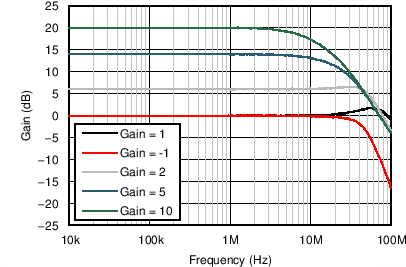VO = 10 mVPP
Figure 2. Small-Signal Frequency Response for Various Gains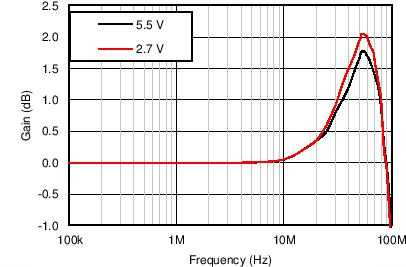VO = 10 mVPP, G = 1
Figure 4. Small-Signal Frequency Response for Various Power Supply Voltages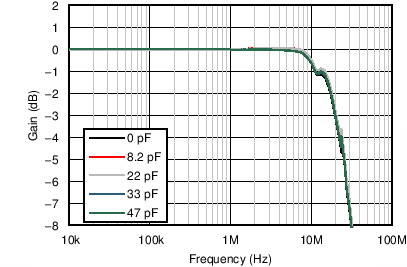VO = 2 VPP, G = 1
Figure 6. Large-Signal Frequency Response for Various Capacitive Loads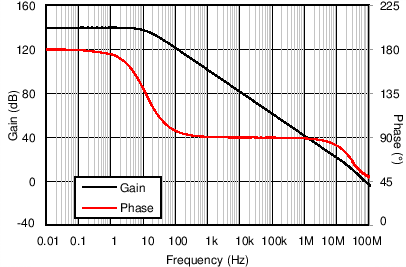VO = 10 mVPP
Figure 8. High-Drive Mode Open-Loop Gain and Phase vs Frequency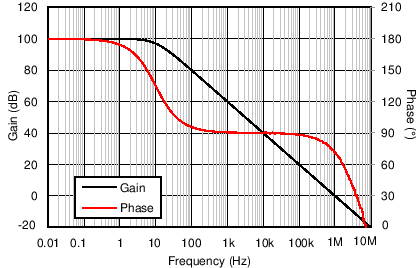VO = 10 mVPP , VMODE = 5 V
Figure 10. Low-Power Mode Open-Loop Gain and Phase vs Frequency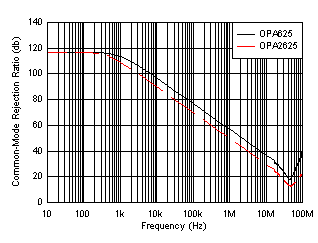Figure 12. Common-Mode Rejection Ratio vs Frequency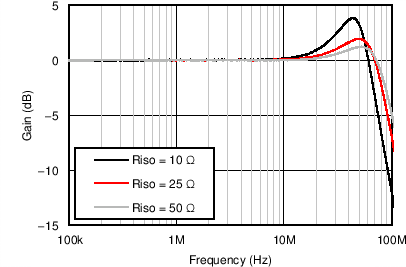G = 1, CLOAD = 1.2 nF
Figure 14. Series Resistance for Capacitive Load Stability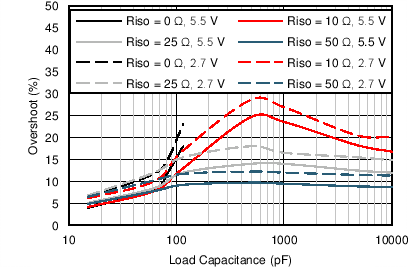G = –1, VO = 10 mVPP
Figure 16. Overshoot vs Capacitive Load, G = –1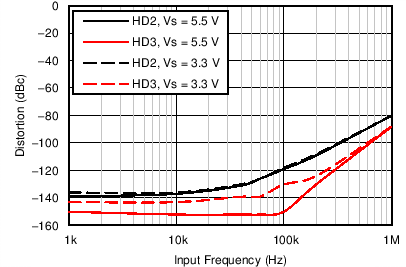G = 1, VO = 2 VPP, RLOAD = 600 Ω
Figure 18. Distortion vs Frequency for Various Power SuppliesG = 1, RLOAD = 600 Ω
Figure 20. Total Harmonic Distortion vs Output Voltage for Various FrequenciesFigure 22. Voltage Noise Density vs Frequency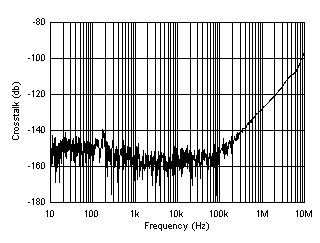Figure 24. Crosstalk vs Frequency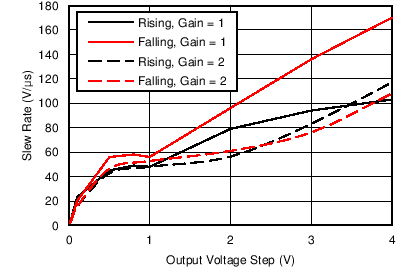Figure 26. Slew Rate vs Output Step Size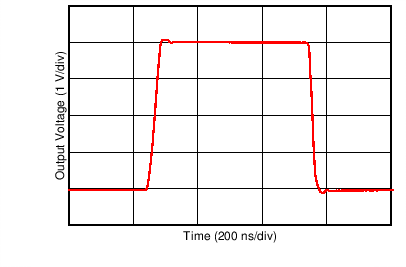G = 1, VO = 4-V step
Figure 28. Large-Signal Pulse Response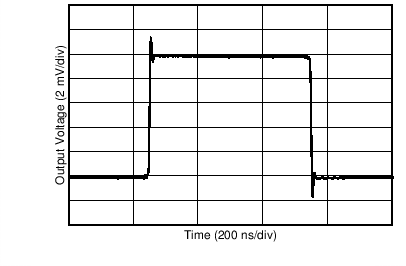G = 1, VO = 10-mV step
Figure 30. Small-Signal Pulse Response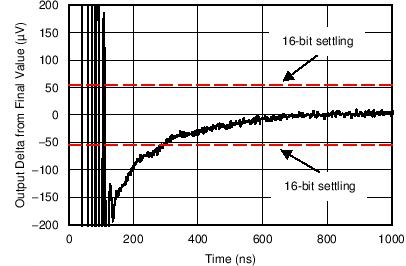VO = 3.6-V step at t = 0 s
Figure 32. 16-Bit Negative Settling Time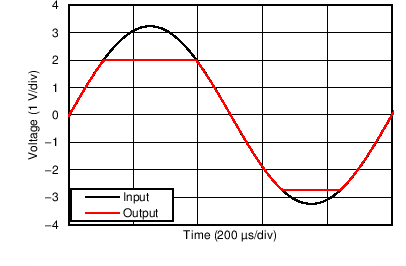VS = ±2.75 V, G = 1
Figure 34. No Phase Reversal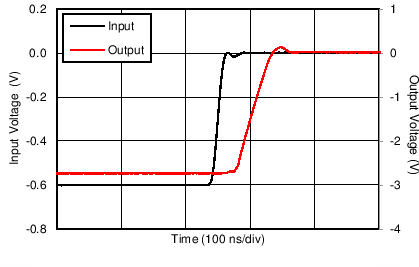VS = ±2.75 V, G = 5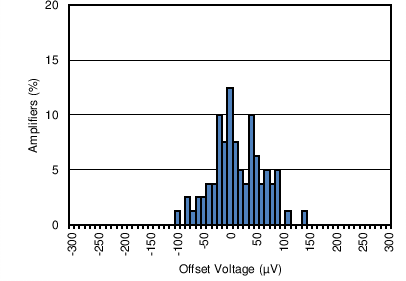Distribution taken from 80 amplifiers, TA = 125°C
Figure 38. Input Offset Voltage Distribution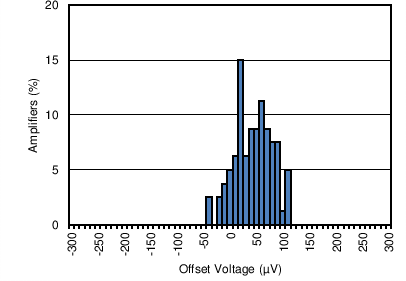Distribution taken from 80 amplifiers, TA = –40°C
Figure 40. Input Offset Voltage Distribution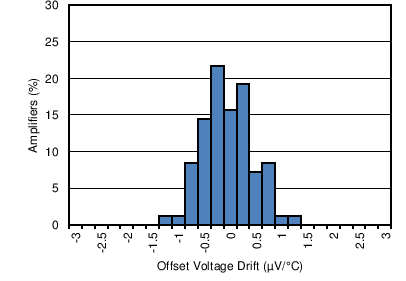Distribution taken from 83 amplifiers, TA = –40°C to +125°C
Figure 42. Input Offset Voltage Drift Distribution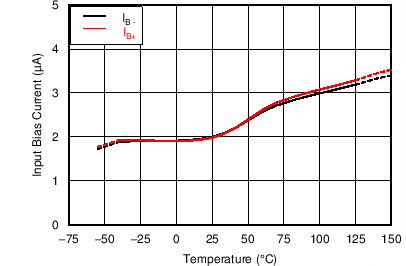Figure 44. Input Bias Current vs Temperature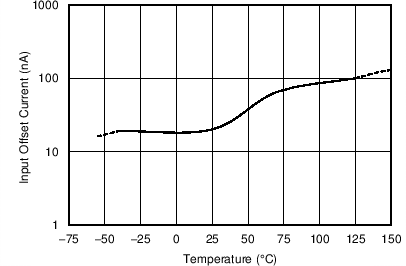Figure 46. Input Offset Current vs Temperature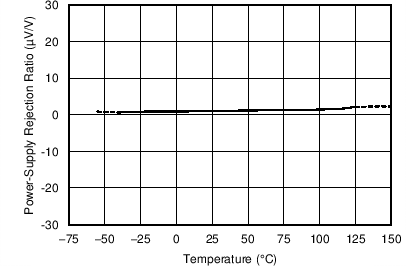2.7 V ≤ VS ≤ 5.5 V
Figure 48. Power-Supply Rejection Ratio vs Temperature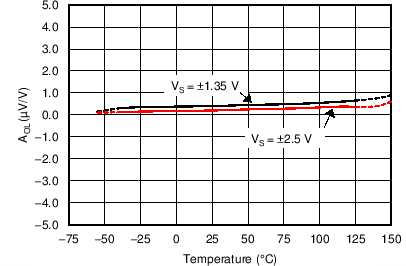Figure 50. Open-Loop Gain vs Temperature with 600-Ω Load6 typical units shown, VS = ±1.35 V to ±2.75 V
Figure 52. Input Offset Voltage vs Power-Supply Voltage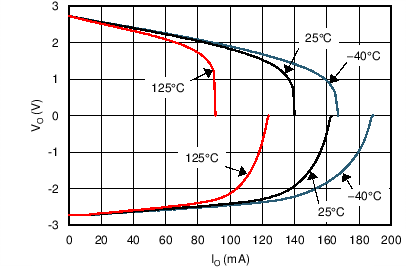Figure 54. Output Voltage vs Output Current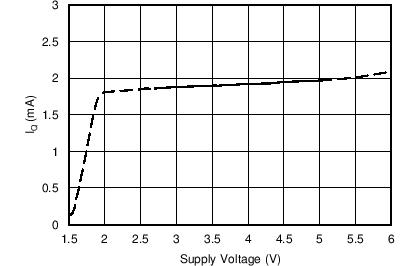High-drive mode
Figure 56. High-Drive Mode Quiescent Current vs Power-Supply Voltage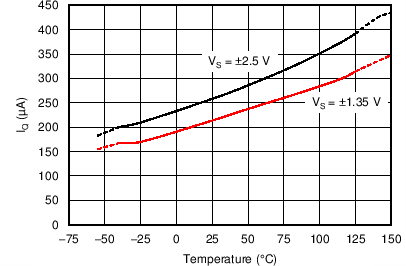MODE pin connected to V+
Figure 58. Low-Power Mode Quiescent Current vs Temperature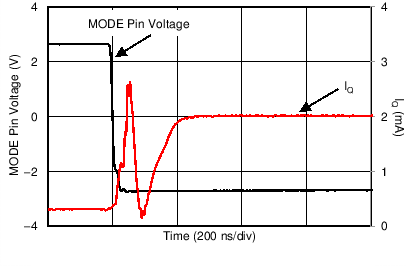VS = ±2.75 V
Figure 60. Quiescent Current When MODE transitions From High To LowVO = 3.8 VDC
Figure 62. Output Voltage When MODE Transitions From High To Low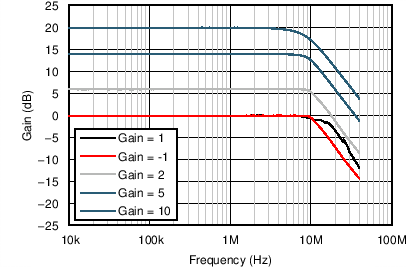VO = 2 VPP
Figure 3. Large-Signal Frequency Response for Various Gains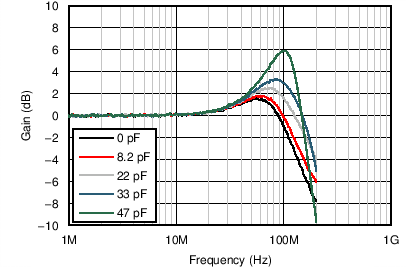VO = 10 mVPP , G = 1
Figure 5. Small-Signal Frequency Response for Various Capacitive Loads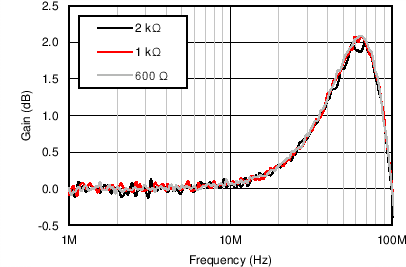VO = 10 mVPP , G = 1
Figure 7. Small-Signal Frequency Response for Various Resistive Loads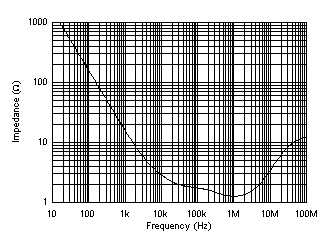Figure 9. High-Drive Mode Open-Loop Output Impedance vs Frequency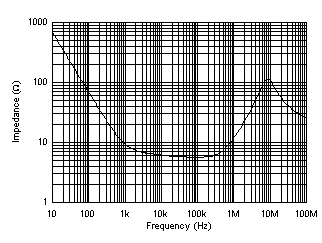Figure 11. Low-Power Mode Open-Loop Output Impedance vs Frequency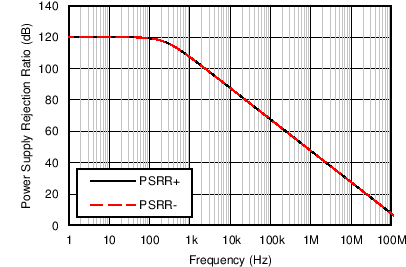Figure 13. Power-Supply Rejection Ratio vs Frequency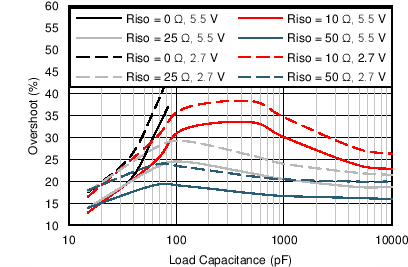G = 1, VO = 10 mVPP
Figure 15. Overshoot vs Capacitive Load, G = 1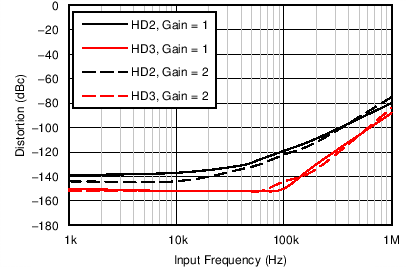VS = 5.5 V, VO = 2 VPP, RLOAD = 600 Ω
Figure 17. Distortion vs Frequency for Various Gains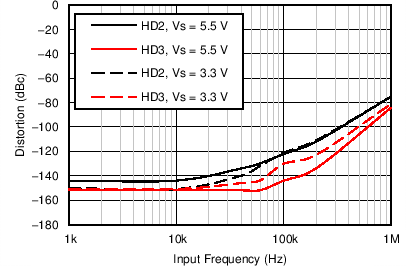G = 2, VO = 2 VPP, RLOAD = 600 Ω
Figure 19. Distortion vs Frequency for Various Power Supplies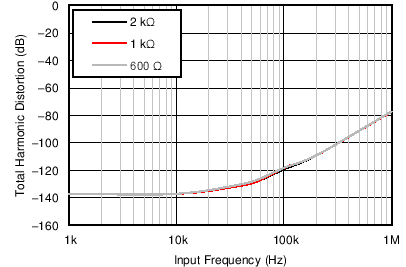G = 1, VO = 2 VPP , RLOAD = 600 Ω
Figure 21. Total Harmonic Distortion vs Frequency for Various Loads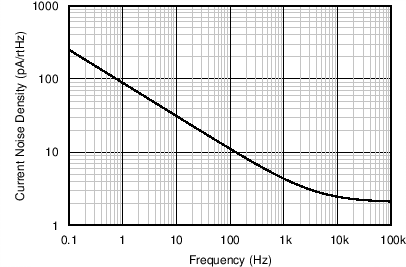Figure 23. Current Noise Density vs Frequency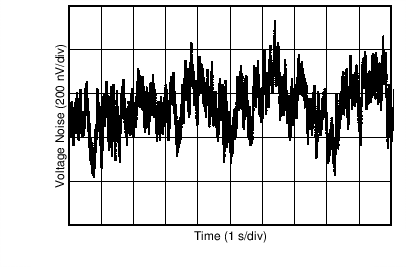Figure 25. 0.1-Hz to 10-Hz Voltage NoiseFigure 27. Maximum Output Voltage vs Frequency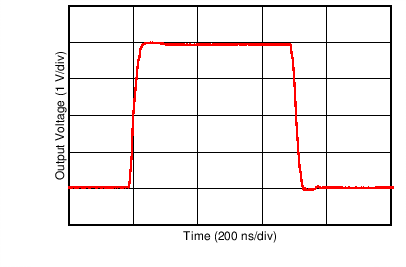G = –1, VO = 4-V step
Figure 29. Large-Signal Pulse Response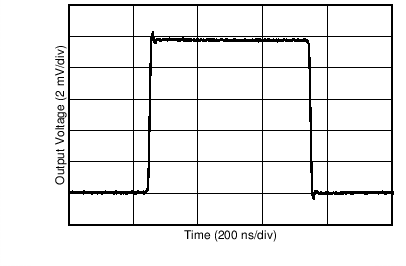G = –1, VO = 10-mV step
Figure 31. Small-Signal Pulse Response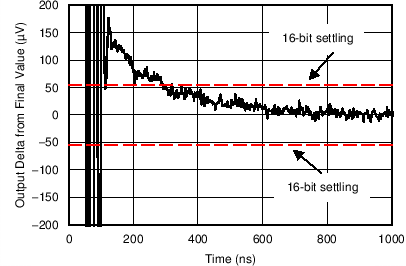VO = 3.6-V step at t = 0 s
Figure 33. 16-Bit Positive Settling Time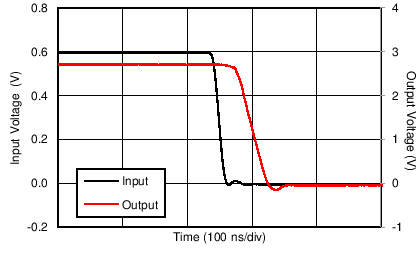VS = ±2.75 V, G = 5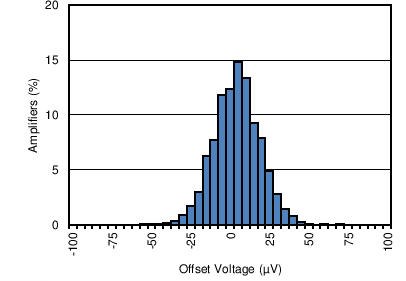Distribution taken from 3139 amplifiers
Figure 37. Input Offset Voltage Distribution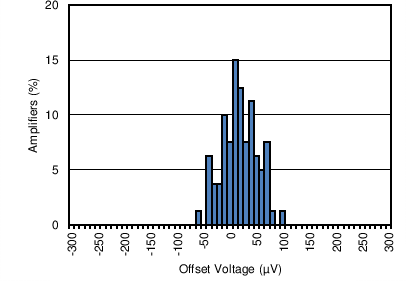Distribution taken from 80 amplifiers, TA = 85°C
Figure 39. Input Offset Voltage Distribution7 typical units shown
Figure 41. Input Offset Voltage vs Temperature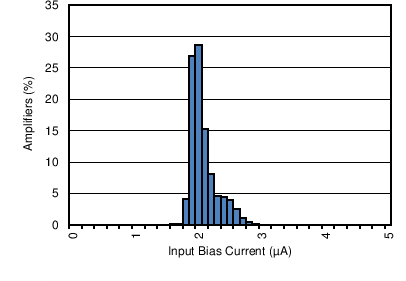Distribution taken from 3139 amplifiers
Figure 43. Input Bias Current DistributionDistribution taken from 3139 amplifiers
Figure 45. Input Offset Current Distribution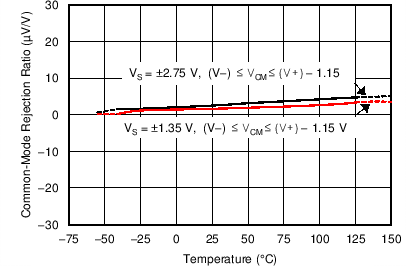Figure 47. Common-Mode Rejection Ratio vs TemperatureFigure 49. Open-Loop Gain vs Temperature with 10-kΩ Load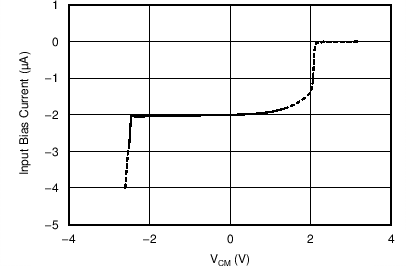High-drive mode, VS = ±2.5 V
Figure 51. Input Bias Current vs Input Common-Mode Voltage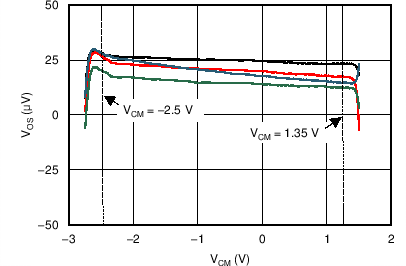6 typical units shown, VS = ±2.5 V
Figure 53. Input Offset Voltage vs Common-Mode VoltageFigure 55. Short-Circuit Current vs Temperature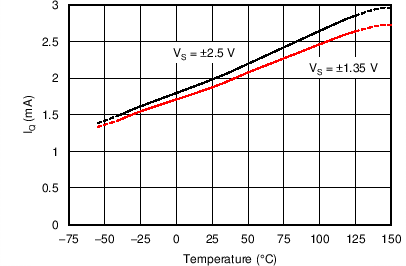Figure 57. High-Drive Mode Quiescent Current vs Temperature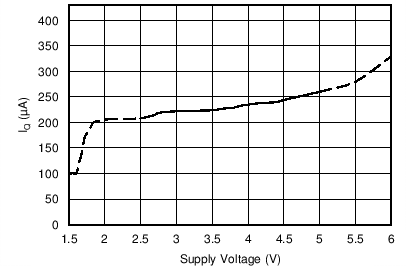Low-drive mode, MODE pin connected to V+
Figure 59. Low-Power Mode Quiescent Current vs Power-Supply Voltage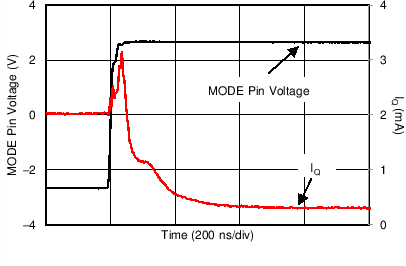VS = ±2.75 V
Figure 61. Quiescent Current When MODE Transitions From Low To High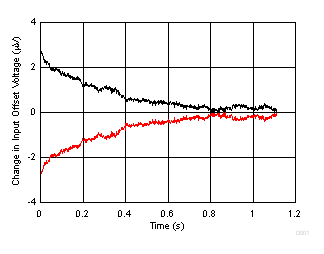OPA625 powered on in high-drive mode at t = 0 s, PCB dimensions: 4 in2, 2 layer, FR4
Figure 63. Warm-Up Time